# Introduction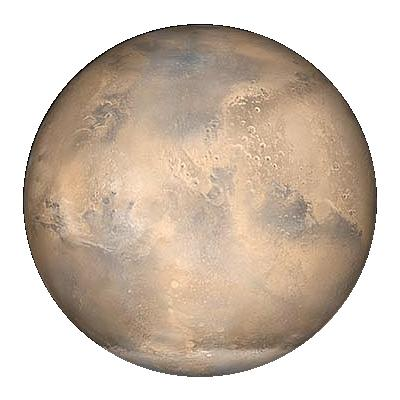With the increasing rate of exploration of Mars continues, data rates from Mars to Earth and back will exceed the current capacity of the DSN to probe links. Furthermore, connections to Mars terminals cannot be maintained throughout the Martian day due the obstruction of the planet itself. To alleviate the bandwidth and outage problems, MarsNet proposes a system of relay satellites orbiting Mars that handle the Earth to Martian communication.

This proposal gives the high-level overview and technical details for developing and deploying the MarsNet Martian-Aresian Relay System (MARS). In Section 1, we document the known, relevant information about. In Section 2, an overview of the system is given with the link budgets following in Section 3. In Section 4, we describe the channel access scheme, the modulation scheme, and the dynamic code allocation protocol. A detailed communication systems diagram is given in Section 5, detailing the receiving and transmitting blocks for each component in the system. Costs analysis and deployment schedule is given in the last section, Section 6.

Please note that there are many images that have been scaled down for screen space. You can click on the image for their full size and click again to return them to the smaller image.

# 1. Mars Overview

In order to reacquaint you with the specifics of Mars, we provide here a quick overview of the properties of Mars. We start with the properties of the planet itself, and then put everything in focus with the solar orbits (ephemeris). Lastly we provide charts of distances and angles between Earth and Mars.

## 1.1 Planetary Specifics

Mars has about a tenth of the mass as Earth but is only half the diameter of the Earth. The surface gravity is only a third of Earths'. The black-body temperature in Kelvin is 210.1 degrees. We will use this value in many of our noise calculations. There are two natural satellites, Deimos and Phobos.
Mar's atmosphere has approximately 7 millibars of pressure with a scale hight of 11.1 km. Mars commonly hash winds from 2 to 10 m/s with 30 m/s winds possible during dust storms. The atmosphere contains about 1/1,000 of the water vapor that Earth does and precautions should be made to avoid transmissions in the 22.2 GHz range as the water-vapor resonance would absorb the propagated power.

## 1.2 Ephemeris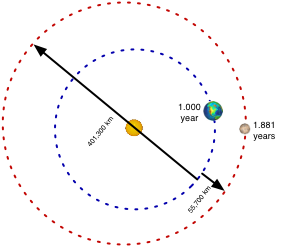Mars' rotational period of 24.5 hours is slightly longer than that of Earth, but its revolutionary period is nearly twice totaling 686.98 days. The orbital inclination from Earth's orbital plane is 1.850 degrees. Approximately every 780 days, the Earth makes a straight line through the Sun to get to Mars. This is termed the Mars-Earth Solar Conjunction. Also, Earth's moon can block the direct line of sight (LOS) to Mars for short periods each month. The use of a solar orbiting satellite with the same elliptical as Mars but a different orbitial angle, could facilitate communication during these month-long periods of conjunction as well as the monthly occlusions by the moon.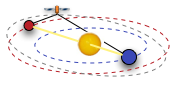## 1.2.1 Earth-Mars Distance

To plan for changing path loss and to calculate the expected Doppler shifts, the distance between Earth and Mars was plotted from Xephem. The data was sampled every fifth date for 31 years. The doppler shift was calculated based on a center frequency of 32.05 GHz. The distances range from 55.7 million kilometers to 401.3 million kilometers. This causes the path loss to range from 277 dB to 295 dB. A histogram of the amount of time spent in each dB of path loss is provided below. The average value of the path loss is 289.86 dB. The doppler shift is calculated by (fdop)max = VT/λ.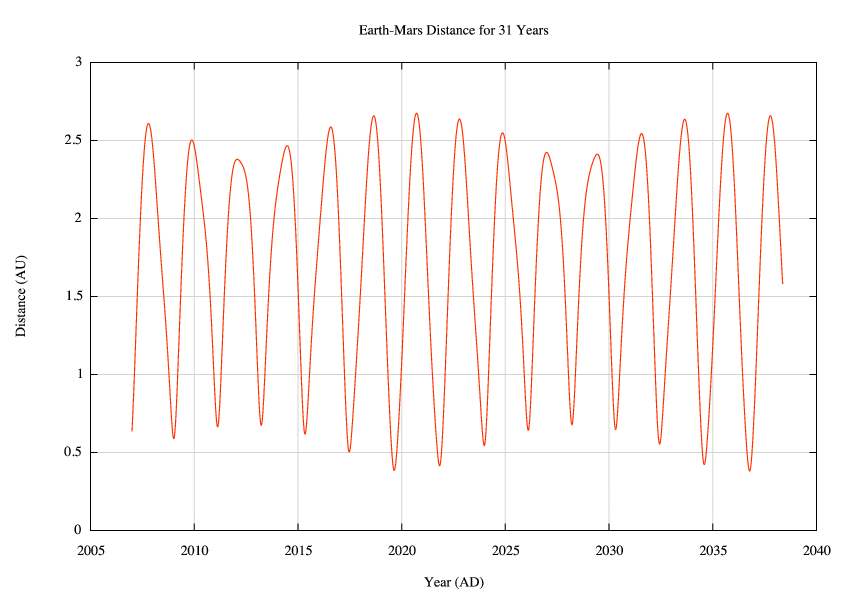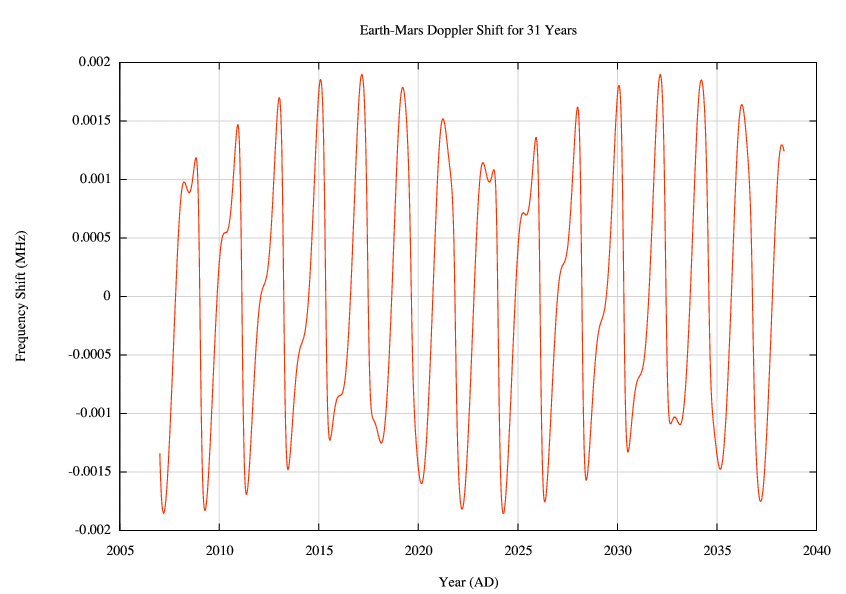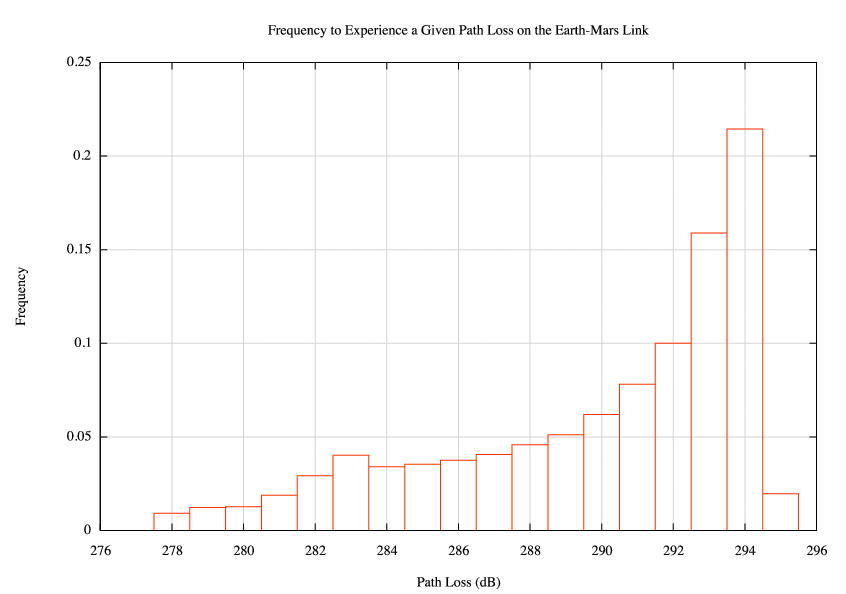# 2. System Overview

The MarsNet Martian-Aresian Relay System (MARS) was designed to provide high speed connections to up to 50 terminal stations located anywhere on Mars. Each station would be able to send up to 1.5 Mbps and receive, maximally, 2 channels of 1.5 Mbps each. One of the two channels would be from Earth and the other would be from another mars terminal. The data stream must allow for a full capacity load at 75 Mbps instream and outstream from Earth. The relay satellites will be capable of receiving 50,1.5 Mbps streams from Mars terminals and be able to send 100, 1.5 Mbps streams back to mars.

## 2.1 Deep Space Network

The MARS will relay between Earth and Mars via use of Earth's Deep Space Network (DSN). The DSN has three 34-meter antennas, one in California, one in Spain, and one in Australia. For the purposes of this design, we assume the availability of a DSN antenna at all times. The DSN will send at 34.45 GHz and receive at 32.05 GHz.

## 2.2 Satellite Configuration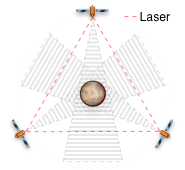In order to fully cover the surface of Mars with minimal cost and power, three satellites and two polar antennas will be deployed to Mars. The satellites will be in an Extremely high Martian Orbit (EMO) at 778,990 km from the center of Mars. This would require a half-power beam width of only 0.5004 degrees using a 5 meter dish to cover the entire side of the planet. Any equatorial orbit would leave the poles uncovered. For that matter, no three satellites can completely cover a sphere. However, cheap, short, passive antennas can be placed at the poles that will relay signals from the uncovered polar region to the satellites. We explain our choice in orbit in Section 3.4.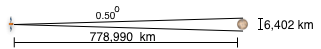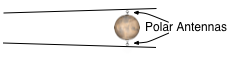The satellites will communicate with each other through low power, high bandwidth lasers. Since the location of each satellite is stationary in repect to the other two satellites, very few adjustments would be needed to maintain the connectivity of laser enabled communications. The laser will operate just under a total energy draw of 1 W per laser.

# 4. Channel Design

The next stage of the design requires the simulateneous access of stations to send and receive information at the same time. CDMA was chosen because it utilizes the bandwidth efficiently, minimizes interference from other terminals, and will allow us to dynamically assign PN codes to nodes as the register and subscribe.

## Multiple Access - CDMA

CDMA operates by multiplying the bits of a signal with a chipping sequence. The chipping sequence chops each bit into M slices, where M is your processing gain. Thus, your chip rate will be M times your bit rate. The chipping sequence is designed so that it looks like noise, has a unit power when correlated with itself, and looks like noise if correlated with another chipping sequence or itself with at least one chip offset in time. To decode a CDMA signal, you need to know the correct code and the correct time offset. Often these codes are found by searching. In MarsNet, we reserve a PN code for registration and subscription and another PN code for replies back to the terminals.

## Code Selection

The MarsNet CDMA system will use the 1024 chips per bit Gold Codes to generate a set of ortagonal codes that can be assigned to terminals as the register and to broadcasts as terminals subscribe to them.

## Traffic Controller

The traffic controller keeps track of where information is coming from and going to and intelligently routes it to all interested interfaces. If the satellite is currently the primary Earth relay, then all information from Earth is routing to the hosting satellites of the information and then they transmit the messages to the mars terminals. Any received terminal information is routed back to Earth. If the satellite is not the primary Earth relay, then it must send all terminal information to the satellite that is the primary relay.
The traffic controller also handles routing subscribed messages to interested satellites and/or terminals. If a terminal on another satellite is subscribed to a broadcast from one of the local satellite's terminals, then the local satellite must forward the traffic to the interested satellite(s). Also, if someone from the local satellite is subscribed to a broadcast on the same satellite, then the broadcast must be routed back down through the Mars antenna. Note that the rebroadcast will likely be on a different PN code than it was originally transmitted with by the terminal.

## Terminal Registration and Subscribing

When a terminal wants access, it sends a registration request containing its unique identifier using the PN code #2. Then it sets a random timer and waits for a reply. The nearest satellite, should negotiate with the other two satellites and agree to host the terminal. Then the satellite will send a registration reply to the terminal on PN code #1 with the terminals unique identifier and two PN code ids (2~37). The terminal will use the first code id as its transmit code and the second as its receiving code. Using this protocol, the satellites can control the use of codes to keep them maximally orthagonal and reused.
When a station (Sue) wants to tune into another station (Stan), Sue sends a subscription request with the unique id of Stan. If the hosting satellite is already routing Stans traffic, it informs Sue of which code to listen to; otherwise, the satellite will request the other satellites to send Stan's broadcast to it, will assign the broadcast a local transmit code and then informs Sue of the local transmit code allocated for Stan's broadcast. Using this method, if multiple terminals want to receive the same broadcast, they simple tune into the same local code.

# 5. Satellite Design

## 5.1 Antennas

The MARS satellites will have two 5 meter antennas, one for communications with Earth and one for communications with Mars. The designed efficiency of the antennas is η = 0.75. The satellites will use lasers to communication amongst themselves.

## 5.2 Power System

The MARS satellites will use nuclear power. Nuclear power is proven itself quite reliable in satellite deployments. Since we are only using 3 satellites, reliability must be ensured. Additionally, the contaimination risk is low due to the limited number of launchings.

## 5.3 Orbital Controls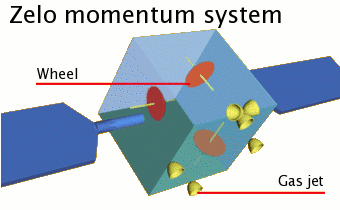The MARS satellites will use the Zelo momentum system to perform orbital corrections. This system uses momentum wheels and gas jets to correct the motion and speed of the satellites. Accurate adjustments of our orbits becomes crutial when using narrow-beam lasers for inter-satellite communication.

## 5.4 Communication Systems

The MarsNet system is comprised of many communications links and as a result has many communication components. In this section we outline the communications system at each of the Earth Station, MARS, and mars terminals. To save time and space, there are 4 building block circuits in use in these diagrams.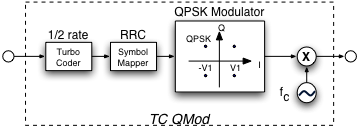The turbo code, QPSK modulator takes in a bitstream, codes it with a 1/2 rate turbo coder, and maps the coded stream using a raised cosine function into symbols for the in-phase and quadrature channels of a π/4 QPSK modulator. The output of the QPSK modulator is then moved into the RF band by modulating the signal with the carrier frequency.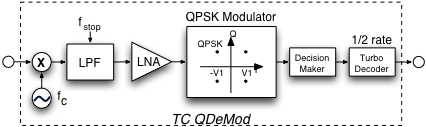The RF signal is first moved to base band and then low pass filtered at the stop frequency. The signal is then amplified with a low noise amplifier and fed into a QPSK demodulator. The output of the demodulator goes into a decision maker, which uses matched filters, to form a bitstream. The bitstream is then decoded by the turbo decoder.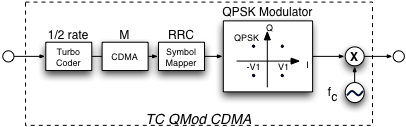Similar to the turbo code, QPSK modulator, this CDMA varient chips the bitstream after the turbo coder has protected the stream.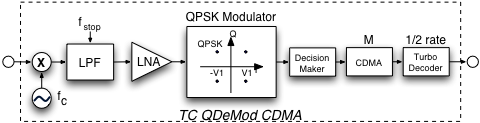The CDMA varient of the decoder tried to chip and correlate the bit stream to decode a channel. The CDMA chip is places after the decision maker and before the turbo coder.

### 5.4.1 Earth DSN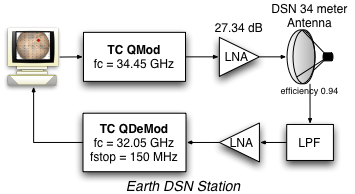At the Earth station, up to 50, 1.5 Mbps streams are muxed together to form a 75 Mbps data stream. The data stream is then sent using the turbo code-QPSK circuit. The pulse shape is a raised cosine with a rolloff factor of k = 0.9. The output RF signal is modulated at 34.45 GHz. The output is amplified by 27.3447 dB and sent out of the 34 meter DSN antenna. The antenna has a gain of 81.5112 dB.
On the receive side, a low pass filter keeps the amplifier in the linear region and removes some bands of noise. The variable amplifier then raises the signal to a level that the TC-QPSK demodulator can use and passes the signal to the demodulator. The output is sent to the monitoring station.

### 5.4.2 MarSat to Earth Systems

The satellite communication system diagrams are separated into pieces to allow better understanding of each piece. There is circuitry to combine the systems together that is rather straightforward and is omitted for clarity.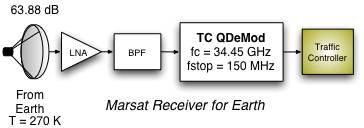The MarSat receives a 34.45 GHz signal from Earth with a 5 meter dish (63.88 dB gain) and has the signal amplified and band-pass filtered. The signal is then reduced to base-band, low-passed, amplified, and demodulated from QPSK. The I and Q channels are deciphered and a bit stream is feed into the turbo coder. The turbo coder sends the corrected bit stream into the traffic controller.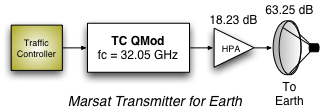The traffic controller send a bit stream into a 1/2 rate turbo coder and then feeds a pulse-shaper to generate a I and Q channel to be feed into the QPSK modulator. The baseband signal is then modulated to the carrier frequency of 32.03 GHz, amplified, and propagated back to Earth.

### 5.4.3 MarSat to MarSat Systems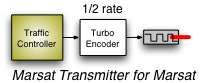The MarSat to MarSat communication is handled almost entirely by the laser. The traffic controller sends a bitstream to the 1/2 rate turbo coder. The turbo coder feed the bitstream into a laser.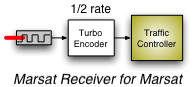The laser detects bits and sends the bitstream to the turbo decoder. The turbo decoder corrects the stream and sends it to the traffic controller.

### 5.4.4 MarSat to Mars Systems

The MarSat to Mars communication link uses CDMA to reduce the interference from ongoing communications. Additionally it allows efficient reuse of the spectrum by allowing signals orthongonal in code to be transmitted at the same time.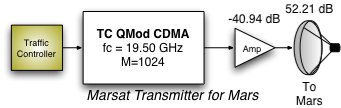The traffic controller codes the bits and then performs CDMA on the bits to spread the sprectrum. The TC-QPSK modulator then modulates the bits into the RF waveform at 19.0 GHz. Note that this process is done for each channel that the satellite transmits and the result is added together before the amplifier.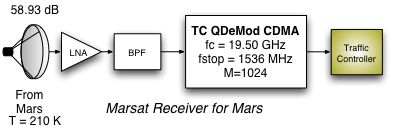The MarsSat receives from Martian terminals and performs a low noise amplification, a bandpass filtering and then decodes with the TC-QPSK demodulator. At the CDMA stage, the satellite will try to correlate the bits for all the registered transmitters for their codes plus the PN code #2 (used for registrations). All of the resulting bitstreams are decoded and passed to the traffic controller.

### 5.4.5 Mars Terminal Systems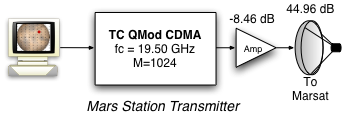The mars terminal transmitter passes its bitstream to the TC-QPSK modulator using its assigned transmit code. The resulting waveform is sent then amplified and sent to the marsat.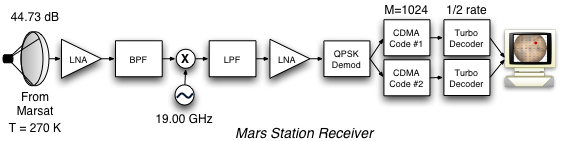The terminal station first amplifies the signal, performs a bandpass, demodulates the signal into baseband, performs a low pass filter, and performs a QPSK demodulation (and decision making). The bitstream is then checked for messages with two PN codes, one assigned as the station's receive code, and the other assigned as the code for the subscribed broadcast.

# 6. Cost Analysis

Each satellite costs approximately \$200 million to build and launch and \$1 million per watt to power. The MarsNet solution minimizes both the number of satellites and the power transmitted by each satellite. The cost break down is as follows:
ItemCountCostTotal (Millions)
Satellite3\$200\$600.00
MarSat-Earth65.0115 Watts x 3 Sats\$1/Watt\$195.03
MarSat-MarSat1 Watt x 6 Lasers\$1/Watt\$6.00
MarSat-Mars0.00010740 x 3 Sats\$1/Watt\$0.00
Polar Antennas2 Antennas\$100/Antenna\$200.00
Total\$1001.00

## 6.1 Satellite Parts

Module Part # Features Cost
Turbo Code ComBlock COM-7001 Turbo-Code Error Correction Demodulator

Rate 0.25 to 0.97

Block Length: 64 bits to 4K bits

\$375

Low Noise Amplifier Northrop Grumman ALH403

27dB gain

61K Noise Temp @ 500MHz

Bandpass Filter Wenzel Electronics Low Noise Bandpass Filter Noise Temp: 36.214 @ 500 MHz

\$44

Local Oscillator Epson EG-2121CA

Low Jitter, High-frequency oscillator

Accuracy: 50 ppm

\$54
PN Code / BPSK Demodulator COM-1011 Direct Sequence Spread-Spectrum Demodulator

Built in BPSK/QPSK demodulator

\$295
Dish Antenna Harris Satellites Dish Antenna

4m diameter dish

50.2534 dBi gain @ 8.425 GhZ

# A. Source Code

## Orbit Optimization

```G = 6.67300E-11;
k = 1.3806503E-23;
AU = 149597870691;
c = 299792458;
DAY = 24*60*60;

mars_mass = 6.4185E23;
mars_rotation = 1.025957*DAY;
mmo = ( ( sqrt( G * mars_mass ) * mars_rotation ) / ( 2 * pi ) )^(2/3);

ff = 19E9;
rr = logspace( 1, 10, 3000 );
ll = 3E8./ff;
Pr = -129.36;          %this is set to the noise power to make the C/N = 0
mr = 3402E3;           % radius of mars
mu = 42828E9;          % the GMp product
v = sqrt(mu./(rr+mr)); % the velocity of the satellite at altitude rr

eff = 0.75;            % antenna efficiency

% I always wanted to use the half-power band width that would provide me the
% most surface of the planet.  In section 3.4, there's an illustration of the
% satellite setting its HPBW at the tangent of the planent (point A).
% this equation finds that angle and multiplies it by 2 to get the HPBW
hpbwr = 2*asin(mr./(rr+mr));  % HPBW in radians
hpbwd = rad2deg(hpbwr);       % HPBW in degrees

% this is the worst case doppler shift (actually beyond worst case)
dop = (1./ll).*(v.*sin(hpbwr./2) - (2*pi*mr/mars_rotation));

% gain of the transmitter based on the HPBW
Gt = lin2db(55517*eff ./ (hpbwd.^2));
% gain of the receiver based on the frequency
Gr = lin2db(eff*pi^2 ./ ll.^2);
% the diameter of the transmitting antenna
D = 75*ll./hpbwd;
% the height of the polar antenna
A = (rr+mr).*tan(hpbwr/2) - mr;
% index of the optimal value (since I can use a dish larger than 5 meters)
i = max(find(D<5));
% index of the marsynchronous orbit
n = max(find(rr<(mmo-mr)));
% the furthest point of communication at the HPBW line (worse than worse case)
rh = rr./cos(hpbwr/2);
% Path loss based on WtWCS
PL = 2*lin2db(4*pi ./ ll) + 2*lin2db( rh );
% HPBW is 3 dB down, so I need to give them 3 dB more power
HP = 3;
% Required transmit power
Pt = Pr - Gt - Gt - Gr + PL + HP;

text;
semilogx( rr, dop/1E6, '-;Doppler Shift;', \
[mmo-mr-1E-6 mmo-mr+1E-6], [-0.1 0.2], '-k;Marsynchronous;', \
[rr(i)-1E-6 rr(i)+1E-6], [-0.1 0.2], '-k;Optimal Radius;' );
xlabel( 'Distance from Mars' );
ylabel( 'Frequency Shift (MHz)' );
title( 'Doppler Shift vs. Orbit Elevation' );

text;
semilogx( rr, Pt, '-;Transmit Power;', rr, Gt, '-;Transmit Gain;', \
rr, PL, '-;Path Loss;', rr, hpbwd, '-;Half-power Beam Width;', \
rr, D, '-;Dish Size;', \
rr(n), PL(n), '*b;;', \
rr(n), hpbwd(n), '*m;;', \
rr(n), Pt(n), '*r;;', \
rr(i), PL(i), '*b;;', \
rr(i), hpbwd(i), '*m;;', \
rr(i), Pt(i), '*r;;', \
rr(i), Gt(i), '*g;;', \
[mmo-mr-1E-6 mmo-mr+1E-6], [-100 300], '-k;Marsynchronous;', \
[rr(i)-1E-6 rr(i)+1E-6], [-100 300], '-k;Optimal Radius;' );
text( rr(n), -90, 'Marsynchronous Orbit', 'HorizontalAlignment', 'right' );
text( rr(i), -90, '5 Meter Dish Orbit', 'HorizontalAlignment', 'right' );
text( rr(n), PL(n)+10, strcat(num2str(PL(n)),' dB'), 'HorizontalAlignment', 'right' );
text( rr(n), Pt(n)+10, strcat(num2str(Pt(n)),' dB'), 'HorizontalAlignment', 'right' );
text( rr(n), hpbwd(n)+10, strcat(num2str(hpbwd(n)),' degrees'), 'HorizontalAlignment', 'right' );
text( rr(i), PL(i)+10, strcat(num2str(PL(i)),' dB'), 'HorizontalAlignment', 'right', 'FontSize', 8 );
text( rr(i), Pt(i)-10, strcat(num2str(Pt(i)),' dB'), 'HorizontalAlignment', 'right' );
text( rr(i), hpbwd(i)+10, strcat(num2str(hpbwd(i)),' degrees'), 'HorizontalAlignment', 'right' );
text( rr(i), Gt(i)+10, strcat(num2str(Gt(i)),' dB'), 'HorizontalAlignment', 'right' );
xlabel( 'Distance from Mars' );
ylabel( 'dB for power, loss, and gain; Meters for dish size' );
title('Tradeoff of Transmit Power verses Orbit Altitude');
```

## Raised Cosine Development

```bpsec=75E6;
rate=1/2;
fc=34.45E9;
bpsym=2;

allocated = 500E6;
doppler = 2E6;

alpha = 0.1;

f0 = bpsec/(rate * bpsym);
N = 2^9;
t1 = -20/f0;
t2 = -t1;
t = linspace(t1,t2,64);
f = (-N/2:N/2 - 1)/(t2-t1);
x = (sin(2*pi*f0*t)./(2*pi*f0*t)).*(cos(2*pi*alpha*t*f0)./(1-(4*alpha*f0*t).^2)); % raised cosine pulse
axis;
plot(t,x,'-;;');
title('Raised Cosine with a Rolloff Factor of 0.3');
xlabel('time (s)');
ylabel('Amplitude');

X = fft(x,N);
XX = abs(fftshift(X))*(t2-t1)/N;
XX_new = 20*log10(XX/max(XX));
axis( [ -1000 1000 -100 0.2 ] );
plot(f/1E6,XX_new,'-;;'), grid on
xlabel('Frequency (MHz)'), ylabel('Power (dB)');
title('Power Spectral Density of Raised Cosine with a Rolloff Factor of 0.3');
```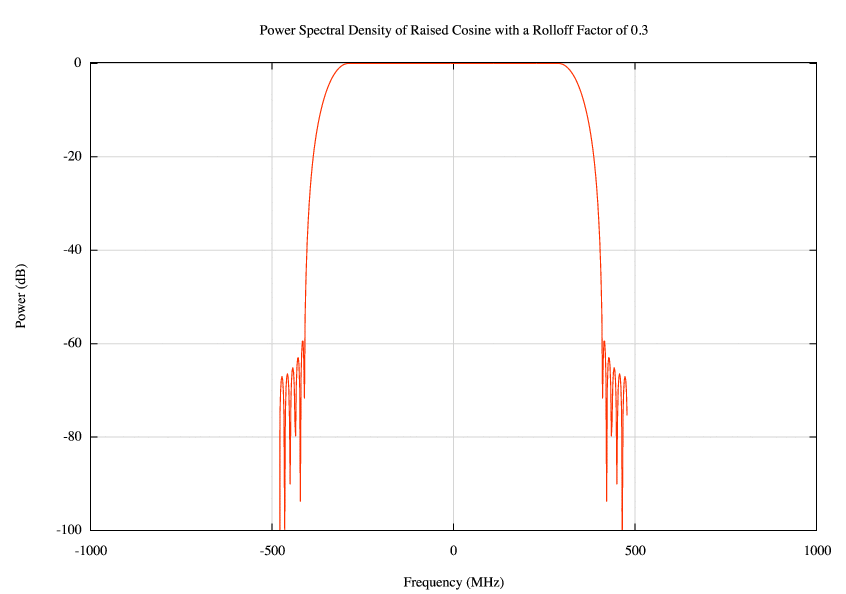## Perl Code

```package MarsSim::Constants;
require Exporter;
@ISA = qw(Exporter);
@EXPORT = qw(M KM AU KG PI DEGREE K SEC MIN HOUR DAY YEAR G LIGHT_YEAR JULIAN_YEAR Hz kHz MHz GHz THz c pi k);

# time in seconds
# temp in kelvin
# mass in kilograms
# length in meters
use constant M => 1;
use constant KM => 1E3;
use constant AU => 149597870.691*KM;
use constant KG => 1;
use constant PI => 3.14159;
use constant DEGREE => 180/PI;
use constant K => 1;
use constant SEC => 1;
use constant MIN => 60;
use constant HOUR => 60*MIN;
use constant DAY => 24*HOUR;
use constant YEAR => 365.25641*DAY;

use constant G => 6.67300E-11; # m3 kg-1 s-2
use constant LIGHT_YEAR => 9.4605284E15*M;
use constant JULIAN_YEAR => 365.25*DAY;

use constant Hz => 1;
use constant kHz => 1E3;
use constant MHz => 1E6;
use constant GHz => 1E9;
use constant THz => 1E12;

use constant c => 299792458;
use constant pi => 3.14159265;
use constant k => 1.3806503E-23; # m2 kg s-2 K-1 (Boltzmann's Constant)

1;
```

```package MarsSim::Antenna;
use Exporter;

@EXPORT = qw(new);

sub new {
my( \$class, %options ) = @_;
die "Cannot create an antenna without a lambda or frequency\n"
unless( defined( \$options{lambda} ) || defined( \$options{frequency} ) );
die "Cannot calculate gain\n"
unless( defined( \$options{gain} ) || defined( \$options{hpbw} ) || defined( \$options{area} ) || defined( \$options{diameter} ) );
\$options{lambda} = c / \$options{frequency} unless defined \$options{lambda};
\$options{efficiency} = 1 unless defined \$options{efficiency};
if( defined( \$options{diameter} ) ) {
\$options{area} = diameter2area( \$options{diameter} );
\$options{gain} = area2gain( \$options{area}, \$options{efficiency}, \$options{lambda} );
\$options{hpbw} = diameter2hpbw(\$options{diameter}, \$options{lambda});#gain2hpbw( \$options{gain} );
} elsif( defined( \$options{hpbw} ) ) {
\$options{gain} = hpbw2gain( \$options{hpbw} );
\$options{area} = gain2area( \$options{gain}, \$options{efficiency}, \$options{lambda} );
\$options{diameter} = area2diameter( \$options{area} );
} else {
\$options{area} = gain2area( \$options{gain}, \$options{efficiency}, \$options{lambda} );
\$options{diameter} = area2diameter( \$options{area} );
\$options{hpbw} = gain2hpbw( \$options{gain} );
}
\$options{temp} = 270*K unless defined \$options{temp};
\$options{bandwidth} = 1 unless defined \$options{bandwidth};
bless \%options, \$class;
return \%options;
}

sub gain {
my( \$self, \$lambda ) = @_;
return( \$self->{efficiency}*4*pi*\$self->{area} / (\$lambda**2) );
}

my( \$self, \$distance, \$dbflag ) = @_;
my \$ll = ( 4 * pi * \$distance ) / \$self->{lambda};
return( (\$dbflag) ? 2*lin2db(\$ll) : \$ll );
}

sub noise {
my( \$self, \$dbflag ) = @_;
my \$n = k*\$self->{tempature}*\$self->{bandwidth};
return( (\$dbflag) ? lin2db(\$n) : \$n );
}

sub hpbw2gain { return( 33000 / (shift()**2) ); }
sub gain2hpbw { return( sqrt(33000 / shift()) )}
sub diameter2area { return( pi*((shift()/2)**2) ); }
sub area2diameter { return( 2*sqrt(shift()/pi) ); }
sub area2gain {
my( \$area, \$eff, \$lambda ) = @_;
return( \$eff*4*pi*\$area / (\$lambda**2) );
}
sub gain2area {
my( \$gain, \$eff, \$lambda ) = @_;
return( \$gain * (\$lambda**2) / (\$eff * 4 * pi) );
}

sub diameter2hpbw {
my( \$dia, \$lambda ) = @_;
return( 75*\$lambda / \$dia );
}

1;
```

```package MarsSim::Planet;

sub new {
my( \$class, %o ) = @_;
bless \%o, \$class;
return \%o;
}

my( \$self ) = @_;
return( ( ( sqrt( G * \$self->{mass} ) * \$self->{rotation_period} ) / ( 2 * pi ) )**(2/3) );
}

1;
```

```package MarsSim::Star;

sub new {
my( \$class, %o ) = @_;
bless \%o, \$class;
return \%o;
}

1;
```

### marsim.pl

```#!/usr/bin/perl -w
use strict;
use Data::Dumper;

galactic_period => 2.26E8*JULIAN_YEAR,
diameter => 1.392E6*KM,
mass => 1.9891E30*KG,
rotation_period => 25.3800*DAY,
);

semimajor => 1.52366231*AU,
eccentricity => 0.09341233,
perihelion => 1.38133346*AU,
orbit_period => 686.9600*DAY,
inclination => 5.65*DEGREE,
eqatorial_diameter => 6804.9*KM,
polar_diameter => 6754.8*KM,
mass => 6.4185E23*KG,
rotation_period => 1.025957*DAY,
axial_tilt => 25.19*DEGREE,
temp_min => 133*K,
temp_mean => 210*K,
temp_max => 293*K,
orbits => \$sun,
);

semimajor => 152097701*KM,
eccentricity => 0.016710150,
perihelion => 147098074*KM,
orbit_period => 365.25641*DAY,
inclination => 7.25*DEGREE,
eqatorial_diameter => 12756.270*KM,
polar_diameter => 12713.500*KM,
mass => 5.9736E24*KG,
rotation_period => 23.934*HOUR,
axial_tilt => 23.439281*DEGREE,
temp_min => 185*K,
temp_mean => 287*K,
temp_max => 331*K,
orbits => \$sun,
);

#	orbits => \$mars,
#	eccentricity => 0,
#);

power => 500E3,
diameter => 34,
frequency => 34.45*GHz,
bandwidth => 150*MHz,
efficiency => 0.94,
tempature => 270*K,
location => \$earth,
);
power => db2lin(18.2340),
diameter => 5,
frequency => 32.05*GHz,
bandwidth => 150*MHz,
efficiency => 0.75,
tempature => 28*K,
);
power => db2lin(-40.94),
diameter => 5,
#hpbw => 19,
frequency => 19*GHz,
bandwidth => 3*MHz,
efficiency => 0.75,
tempature => 28*K,
);
power => db2lin(-40),
diameter => 1,
frequency => 19.5*GHz,
bandwidth => 3*MHz,
efficiency => 0.75,
tempature => 210*K,
);

my \$r = 7.7559e+08;
print( "===== Mars Satellite to Earth =====\n" );
snr2( \$marsat, \$dsn, 401.3E9, 0 );
print( "===== Earth to Mars Satellite =====\n" );
snr2( \$dsn, \$marsat, 401.3E9, 0 );
print( "===== Mars Station to Mars Satellite =====\n" );
snr2( \$station, \$marsat2, \$r, 0 );
print( "===== Mars Satellite to Mars Station =====\n" );
snr2( \$marsat2, \$station, \$r, 0 );

sub snr {
my(\$send, \$recv, \$dist)=@_;
my \$pt = \$send->{power};
printf( "Cost per satellite: \\$%0.4f million dollars\n", \$pt + 200 );
my \$e1 = \$send->{efficiency};
my \$e2 = \$recv->{efficiency};
#my \$fc = 8.43*GHz; my \$bw = 60*MHz;
my \$fc = \$send->{frequency};
my \$bw = \$send->{bandwidth};
my \$d1 = \$send->{diameter};
my \$d2 = \$recv->{diameter};
my \$d = \$dist;
my \$t = \$send->{tempature};
my \$l = c / \$fc;
print( "SNR = Pt + Gt + Gr - PL - Pn\n" );
my \$Pt = lin2db( \$send->{power} );
my \$Gt = lin2db( \$e1 * ((pi*\$d1)**2) / (\$l**2) );
my \$Gr = lin2db( \$e2 * ((pi*\$d2)**2) / (\$l**2) );
my \$PL = 2*lin2db( \$fc*4*pi*\$d/c );
my \$Pn = lin2db( k*\$t*\$bw );
printf( "Pt = %0.4f\n", \$Pt );
printf( "Gt = %0.4f\n", \$Gt );
printf( "Gr = %0.4f\n", \$Gr );
printf( "PL = %0.4f\n", \$PL );
printf( "Pn = %0.4f\n", \$Pn );
my \$snr = \$Pt + \$Gt + \$Gr - \$PL - \$Pn;
printf( "SNR = %0.4f\n", \$snr );
}

sub snr2 {
my(\$send, \$recv, \$dist, \$snr)=@_;
my \$pt = \$send->{power};
my \$e1 = \$send->{efficiency};
my \$e2 = \$recv->{efficiency};
#my \$fc = 8.43*GHz; my \$bw = 60*MHz;
my \$fc = \$send->{frequency};
my \$bw = \$send->{bandwidth};
my \$d1 = \$send->{diameter};
my \$d2 = \$recv->{diameter};
my \$d = \$dist;
my \$t = \$send->{tempature};
my \$l = c / \$fc;
print( "Pt = SNR - Gt - Gr + PL + Pn\n" );
my \$Gt = lin2db( \$e1 * ((pi*\$d1)**2) / (\$l**2) );
my \$Gr = lin2db( \$e2 * ((pi*\$d2)**2) / (\$l**2) );
my \$PL = 2*lin2db( \$fc*4*pi*\$d/c );
my \$Pn = lin2db( k*\$t*\$bw );

printf( "SNR = %0.4f\n", \$snr );
printf( "Gt = %0.4f\n", \$Gt );
printf( "Gr = %0.4f\n", \$Gr );
printf( "PL = %0.4f\n", \$PL );
printf( "Pn = %0.4f\n", \$Pn );
my \$Pt = \$snr - \$Gt - \$Gr + \$PL + \$Pn;
printf( "Pt = %0.4f\n", \$Pt );
printf( "Cost per satellite: \\$%0.4f million dollars\n", db2lin(\$Pt) + 200 );
}
```

# B. Mars Fact Sheet

## Bulk Properties

PropertyMarsEarthRatio (Mars/Earth)
Mass (1024 kg)0.641855.97360.107
Volume (1010 km3)16.318108.3210.151
Ellipticity (Flattening)0.006480.003351.93
Mean density (kg/m3)393355150.713
Surface gravity (m/s2)3.719.800.379
Surface acceleration (m/s2)3.699.780.377
Escape velocity (km/s)5.0311.190.450
GM (x 106 km3/s2)0.042830.39860.107
Bond albedo0.2500.3060.817
Visual geometric albedo0.1500.3670.409
Visual magnitude V(1,0)-1.52-3.86-
Black-body temperature (K)210.1254.30.826
Topographic range (km)30201.500
Moment of inertia (I/MR2)0.3660.33081.106
J2 (x 10-6)1960.451082.631.811
Number of natural satellites21
Planetary ring systemNoNo

## Orbital Parameters

PropertyMarsEarthRatio (Mars/Earth)
Semimajor axis (106 km)227.92149.601.524
Sidereal orbit period (days)686.980365.2561.881
Tropical orbit period (days)686.973365.2421.881
Perihelion (106 km)206.62147.091.405
Aphelion (106 km)249.23152.101.639
Synodic period (days)779.94--
Mean orbital velocity (km/s)24.1329.780.810
Max. orbital velocity (km/s)26.5030.290.875
Min. orbital velocity (km/s)21.9729.290.750
Orbit inclination (deg)1.8500.000-
Orbit eccentricity0.09350.01675.599
Sidereal rotation period (hrs)24.622923.93451.029
Length of day (hrs)24.659724.00001.027
Obliquity to orbit (deg)25.1923.451.074

## Natural Satellites

PropertyPhobosDeimos
Semi-major axis* (km)937823459
Sidereal orbit period (days)0.318911.26244
Sidereal rotation period (days)0.318911.26244
Orbital inclination (deg)1.081.79
Orbital eccentricity0.01510.0005